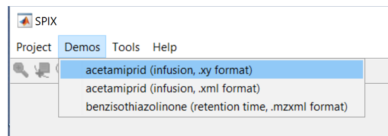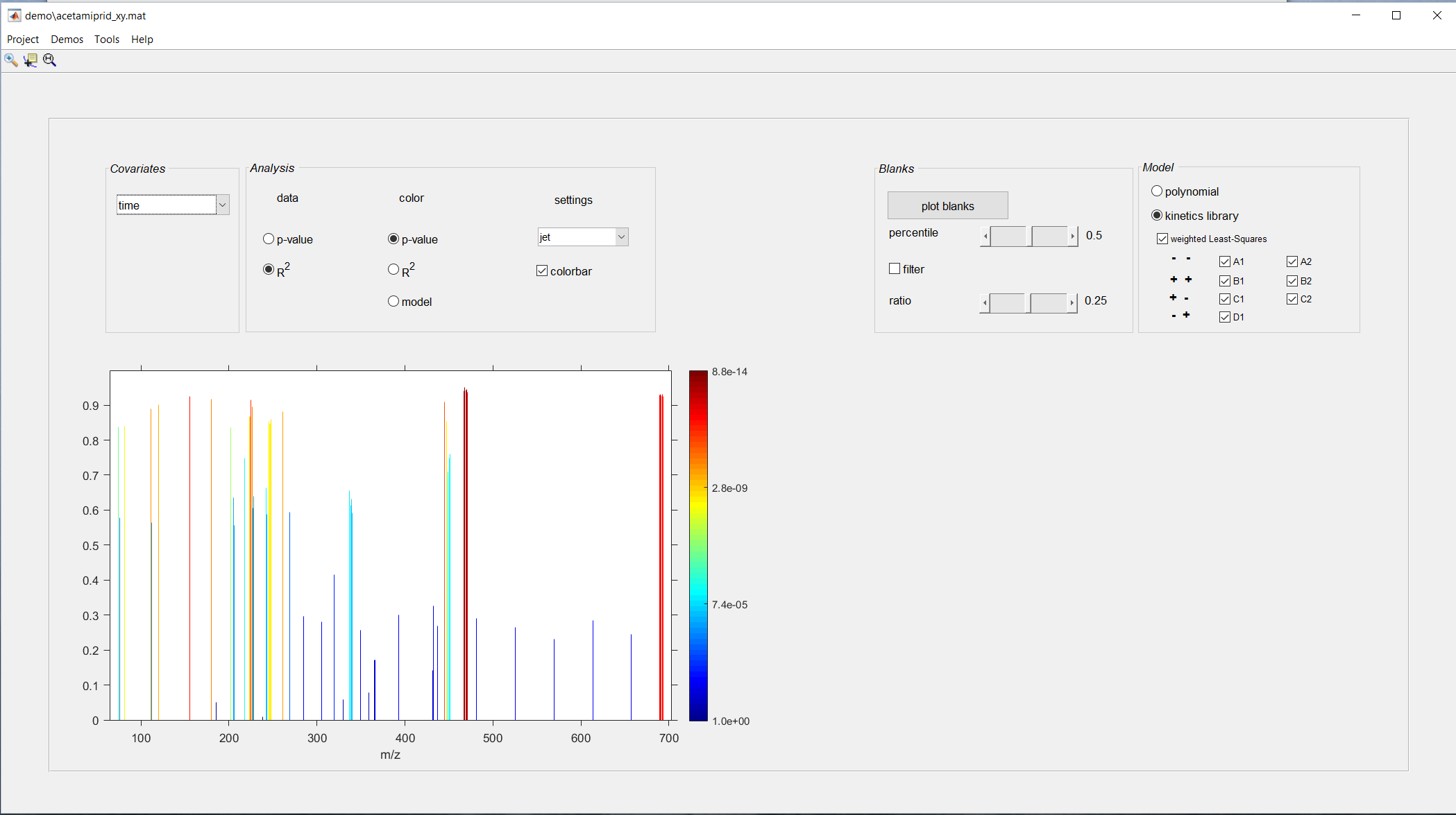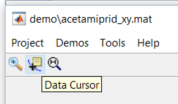This experiment is about the degradation kinetics of a pesticide (acetamiprid) diluted at 10 ppm in water. This degradation is done via a UV lamp to simulate the action of the sun while accelerating the degradation process. The degradation kinetics was performed over 90 minutes in three different tubes (A, B and C) with the following samples for each of them: 0 (reference before photolysis), 1, 2, 5, 10, 30, 60, 90 minutes. The analysis is performed in ultra-high resolution mass spectrometry in positive mode direct injection electrospray using an autosampler. 50 scans are accumulated with a resolution of about 300,000 at m/z 400. Acetamiprid is observed in this study at m/z 223.07 as [MH]+, m/z 245.06 as [MNa]+ and dimers and trimers corresponding to the following m/z: 445.14, 467.12 and 689.19.

# 1 Using the xy format

## 1.1 Run the demo

Select the demo acetamiprid (.xy format)The objective of this study is to see how some peaks of the spectrum evolve over time. The peaks (i.e. the m/z values) associated with a significant change are represented. Here, the part of variance explained by the model is represented on the y-axis while the color represents the p-value.Explore the plotted data by enabling the data cursor modeThen, select a stem (i.e a \(m/z\)) to plot the fitted model and the measured spectra around the spike